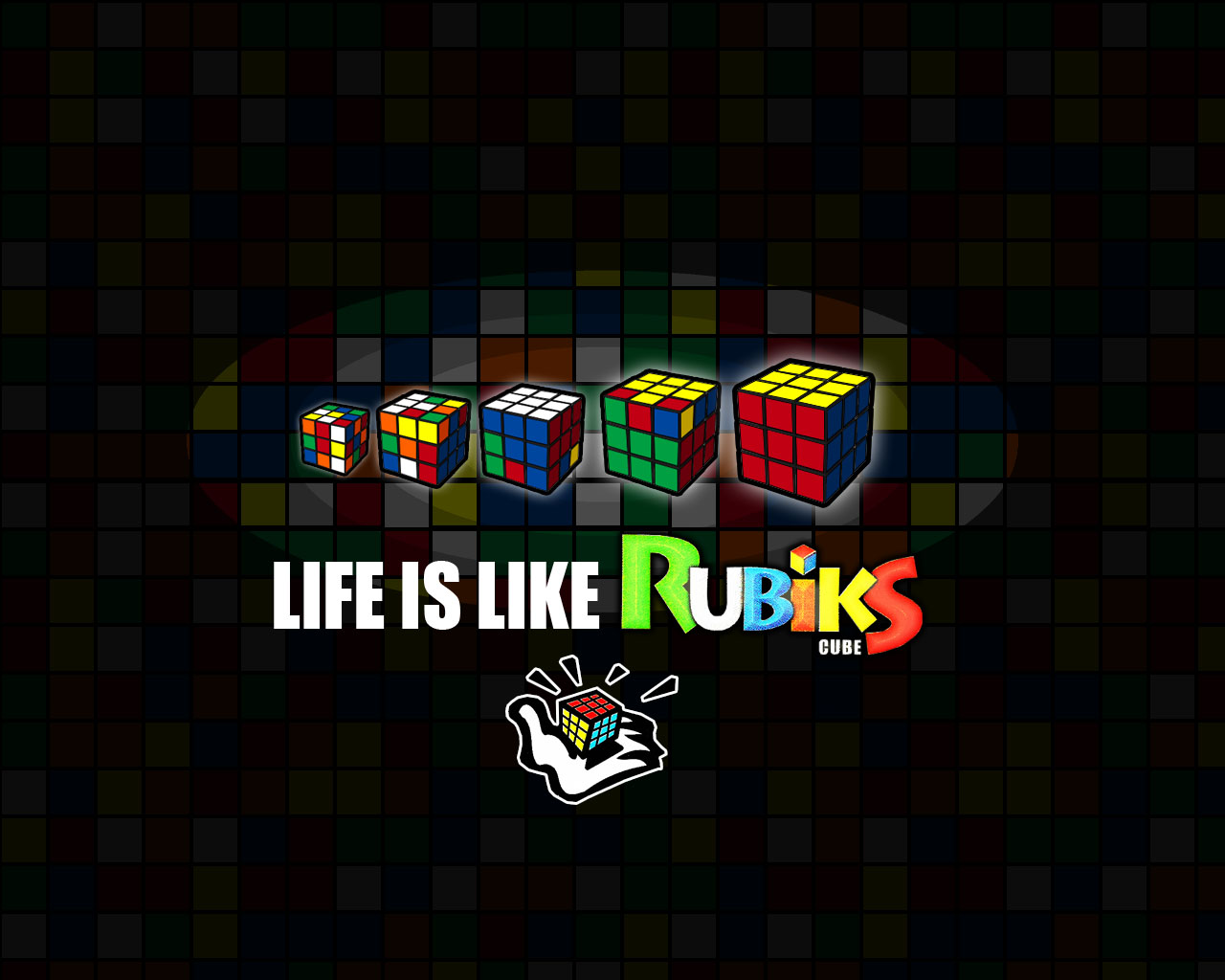# 'Odd' Algorithms

Probability Level 3Consider a Rubik's Cube.

A move is a 90 Degree turn on one of the faces of the cube.

An algorithm is a sequence of moves.

An identity algorithm is an algorithm which when applied on a particular state of the cube results in the exact same state.

How many identity algorithms are there which consist of an odd number of moves?

×

Problem Loading...

Note Loading...

Set Loading...